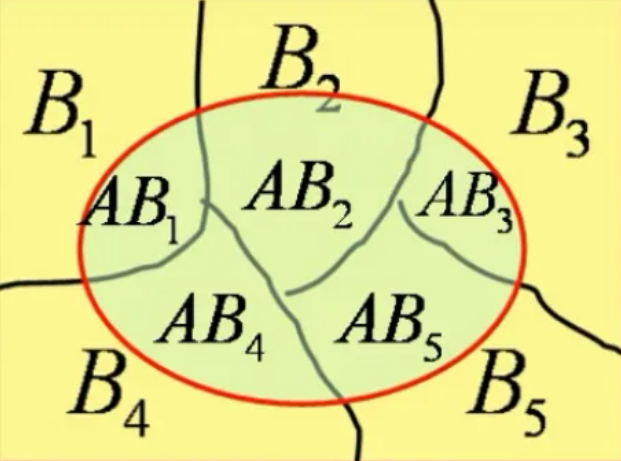1. 确定要求的事件A
2. 找完备事件组，列出各种情况Bi(i=1,2,3...)
3. 在情况Bi下，事件A发生的概率，P(A) = ∑P(Bi)P(A|Bi) = ∑P(ABi)# 分布函数

注意

# 联合概率

🔺注意：不管积分区域是什么样，也就是 P{} 括号内的函数是什么样，和联合分布函数无关。联合分布函数都是 f(x, y) （被积函数）

1. xy都是离散型，很简单，直接看就行
2. xy都是连续型，则把 z 看作常数，讨论 z 的范围，然后再用上面联合概率密度求概率的方法
3. x是离散型，y是连续型（离连型）全概率公式+分布函数法
先写出 P{ X + Y ≤ Z } ，然后用全概率公式，分为 P{ X + Y ≤ Z ，X=0} P{ X + Y ≤ Z ，X=1} 等区间
然后带入 X=0，X=1，化简表达式，变成 P{ X=0，Y ≤ Z }，P{ X=1，Y ≤ Z-1 }
然后讨论 z 的范围，根据 X+Y=Z 来估计。比如 0<X<1，0<Y<1，那么 讨论 Z ∈（0，2）比如 z<0 时，Fz(z)=0
当 z 的取值在积分区域里时，用二重积分计算概率值

# 数字特征

X Y 不相关 ⇔ EXY = EXEY (ρ=0)
X Y 独立 ⇔ FXY = FXFY 或者 f(xy) = f(x)f(y)